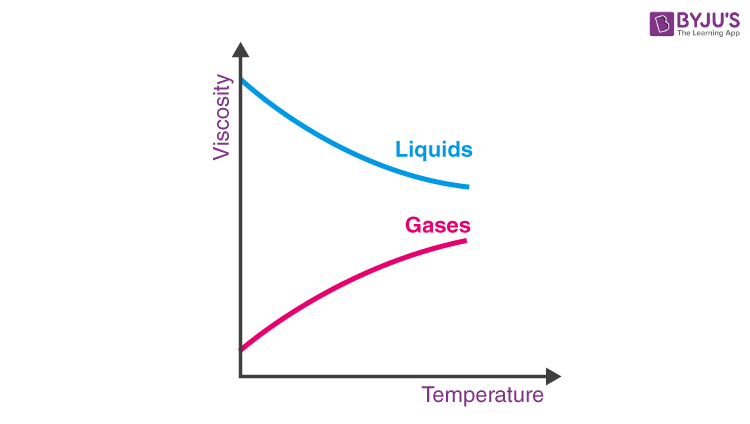# Properties of Fluids

To understand the different properties of fluids, first, we have to understand what exactly is meant by the term fluids. By definition, anything that can flow is fluid. The water we drink, and the air we breathe are all examples of fluids. Essentially, all liquids and gases are fluids. In this article, let us learn about the properties of fluids in detail.

## Different Properties Of FluidsThough each fluid is different from others in terms of composition and specific qualities, there are some properties which every fluid shares.

These properties can be broadly categorized under:

• Kinematic properties: These properties help in understanding the fluid motion. Velocity and acceleration are the kinematic properties of the fluids.
• Thermodynamic properties: These properties help in understanding the thermodynamic state of the fluid. Temperature, density, pressure, and specific enthalpy are the thermodynamic properties of fluids.
• Physical properties: These properties help in understanding the physical state of the fluid such as colour and odour.

Watch the video below to understand the mechanical properties of fluids.### Density

The density of a fluid is defined as the ratio of the mass of the fluid to its volume. The density of gases is dependent on pressure and temperature, while the density of liquid remains constant. The density of water is 1000 kg.m-3 while density of air is 1.225 kg.m-3.

 $$\begin{array}{l}\rho = \frac{mass\;of\;fluid}{volume\;of\;fluid}\end{array}$$

 Density is denoted by ρ SI unit of density kg.m-3

### Specific weight

Specific weight is defined as the weight possessed by the unit volume of a fluid. Specific weight is dependent on acceleration due to gravity as it changes from place to place. The specific weight of water is 9.81 × 1000 N.m-3.

 $$\begin{array}{l}w= \frac{weight}{volume}\end{array}$$

 Specific weight is denoted by w SI unit of specific unit N.m-3

### Temperature

Temperature is one of the thermodynamic properties of fluids that determines the state of hotness or coldness of it. Temperature is measured in either Kelvin or Celsius or Fahrenheit. Kelvin is the most common one that is used because of its independence from the properties of the substance. The following graph clearly depicts the effect of temperature on fluids.### Pressure

The pressure of a fluid is the force applied by it per unit area.

 $$\begin{array}{l}\frac{Force}{Area}\end{array}$$

 Pressure is denoted by p SI unit of pressure N.m-2

### Specific Volume

In fluid mechanics, specific volume is the reciprocal of density. It can be expressed as the volume that a fluid occupies per unit mass.

 $$\begin{array}{l}\frac{Volume}{Mass}\end{array}$$
 Specific volume is denoted by v SI unit of specific volume m3.kg-1

### Surface tension

Surface tension is defined as the phenomenon in which the surface of the liquid is in contact with another phase. The surface tension of water at 100°C is 0.059 N.m-1 and at 0°C it is 0.079 N.m-1.

 $$\begin{array}{l}T=\frac{F}{L}\end{array}$$

 CGS unit of surface tension dyn.cm-1 SI unit of surface tension N.m-1## Frequently Asked Questions – FAQs

Q1

### What is fluid?

Anything that has the property to flow categorizes as fluid.
Q2

### What are the types of matter enfolded under fluids?

Liquids and gases are enfolded under fluids
Q3

### Give an example for fluids.

Examples of fluids are Water, Oxygen, Molten lava, etc.
Q4

### Name the properties of fluids.

There are three properties of fluids. Namely, Kinematic, Thermodynamic and Physical properties
Q5

### Name the Kinetic property of a fluid.

Kinematic properties such as the velocity and acceleration.
Q6

### Name the Thermodynamic property of a fluid.

Thermodynamic properties of fluids are density, temperature, internal energy, pressure, specific volume and specific weight.
Q7

### Name the Physical property of a fluid.

Physical properties of fluids such as appearance, colour, and odour.
Q8

### Arrange the following terms in the ascending order of their density: Water, Carbon dioxide, Air, Seawater.

Carbon dioxide
Define the specific volume.
Q9

### Define the specific volume.

Specific volume is expressed as the volume that a fluid occupies per unit mass.
Q10

### What is the relation between specific volume and density?

Specific volume is the reciprocal of density.

Hope you have got a brief knowledge of fluids, properties of fluids, parameters affecting the properties of fluids, etc. For a better understanding of thermodynamics do read the related articles and answer the practice questions.

### Related Physics Concepts

Stay tuned with BYJU’S for more such interesting articles. Also, register to “BYJU’S – The Learning App” for loads of interactive, engaging Physics related videos and unlimited academic assistance.

Test your knowledge on Properties Of Fluids